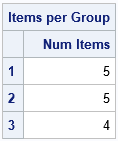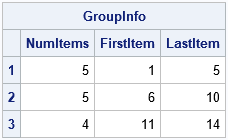Suppose you need to assign 100 patients equally among 3 treatment groups in a clinical study. Obviously, an equal allocation is impossible because the second number does not evenly divide the first, but you can get close by assigning 34 patients to one group and 33 to the others. Mathematically, this is a grade-school problem in integer division: simply assign floor(100/3) patients to each group, then deal with the remainders. The FLOOR function rounds numbers down.

The problem of allocating a discrete number of items to groups comes up often in computer programming. I call the solution the FLOOR-MOD trick because you can use the FLOOR function to compute the base number in each group and use the MOD function to compute the number of remainders. Although the problem is elementary, this article describes two programming tips that you can use in a vectorized computer language like SAS/IML:

• You can compute all the group sizes in one statement. You do not need to use a loop to assign the remaining items.
• If you enumerate the patients, you can directly assign consecutive numbers to each group. There is no need to deal with the remainders at the end of the process.

### Assign items to groups, patients to treatments, or tasks to workers

Although I use patients and treatment groups for the example, I encountered this problem as part of a parallel programming problem where I wanted to assign B tasks evenly among k computational resources. In my example, there were B = 14 tasks and k = 3 resources.

Most computer languages support the FLOOR function for integer division and the MOD function for computing the remainder. The FLOOR-MOD trick works like this. If you want to divide B items into k groups, let A be the integer part of B / k and let C be the remainder, C = B - A*k. Then B = A*k + C, where C < k. In computer code, A = FLOOR(B/k) and C = MOD(B, k).

There are many ways to distribute the remaining items, but for simplicity let's give an extra item to each of the first C groups. For example, if B = 14 and k = 3, then A = floor(14/3) = 4 and C = 2. To allocate 14 items to 3 groups, give 4 items to all groups, and give an extra item to the first C=2 groups.

In a vector programming language, you can assign the items to each group without doing any looping, as shown in the following SAS/IML program:

```/* Suppose you have B tasks that you want to divide as evenly as possible among k Groups. How many tasks should you assign to the i_th group? */ proc iml; /* B = total number of items or tasks (B >= 0, scalar) k = total number of groups or workers (k > 0, scalar) i = scalar or vector that specifies the group(s), max(i)<=k Return the number of items to allocate to the i_th group */ start AssignItems(B, k, i); n = floor(B / k) + (i <= mod(B, k)); /* the FLOOR-MOD trick */ return(n); finish;   /* Test the code. Assign 14 tasks to 3 Groups. */ nItems = 14; nGroups = 3; idx = T(1:nGroups); /* get number of items for all groups */ Group = char(idx); /* ID label for each group */ n = AssignItems(nItems, nGroups, idx); print n[c={"Num Items"} r=Group L="Items per Group"];```The AssignItems function is a "one-liner." The interesting part of the AssignItems function is the binary expression i <= mod(B, k), which is valid even when i is a vector. In this example, the expression evaluates to the vector {1, 1, 0}, which assigns an extra item to each of the first two groups.

### Which items are assigned to each group?

A related problem is figuring out which items get sent to which groups. In the example where B=14 and k=3, I want to put items 1-5 in the first group, items 6-10 in the second group, and items 11-14 in the last group. There is a cool programming trick, called the CUSUM-LAG trick, which enables you to find these indices. The following function is copied from my article on the CUSUM-LAG trick. After you find the number of items in each group, you can use the ByGroupIndices function to find the item numbers in each group:

```/* Return kx2 matrix that contains the first and last elements for k groups that have sizes s, s,...,s[k]. The i_th row contains the first and last index for the i_th group. */ start ByGroupIndices( s ); size = colvec(s); /* make sure you have a column vector */ endIdx = cusum(size); /* locations of ending index */ beginIdx = 1 + lag(endIdx); /* begin after each ending index ... */ beginIdx = 1; /* ...and at 1 */ return ( beginIdx || endIdx ); finish;   /* apply the CUSUM-LAG trick to the item allocation problem */ GroupInfo = n || ByGroupIndices(n); print GroupInfo[r=Group c={"NumItems" "FirstItem" "LastItem"}];```The table shows the number of items allocated to each group, as well as the item indices in each group. You can use the FLOOR-MOD trick to get the number of items and the CUSUM-LAG trick to get the indices. In a vector language, you can implement the entire computation without using any loops.

One more comment: When you run a program in parallel in the iml action, sometimes you want to divide a computation evenly among all available threads. The NPERTHREAD function in the iml action provides this information to each thread. The function uses the local thread ID and the total number of available threads to perform the same computation as the AssignItems function in this article.

Share